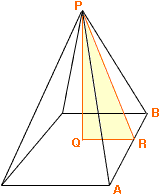Quandaries and Queries Hello I was wondering how to find the area of a pyramid when you don’t know the height of the face but the height of the Pyramids highest point? Jason Hi Jason, If the pyramid is regular then you can do it this way.P is the vertex of the pyramid and R is the midpoint of the side AB. The line PQ is perpendicular to the base so, by the fact that the pyramid is regular, you can conclude that Q is in the center of the square base. Thus |QR| is half the length of AB. PQR is a right triangle and you know |QR| and |PQ| so you can use Pythagoras' Theorem to find |PR|. Penny Go to Math Central